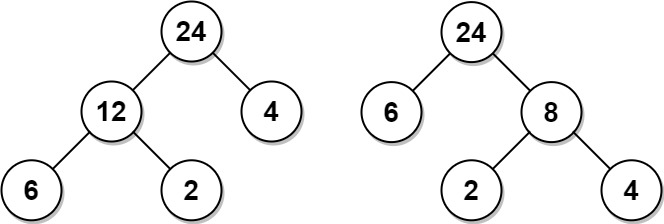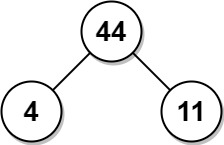## Algorithm

Problem Name: 1130. Minimum Cost Tree From Leaf Values

Given an array `arr` of positive integers, consider all binary trees such that:

• Each node has either `0` or `2` children;
• The values of `arr` correspond to the values of each leaf in an in-order traversal of the tree.
• The value of each non-leaf node is equal to the product of the largest leaf value in its left and right subtree, respectively.

Among all possible binary trees considered, return the smallest possible sum of the values of each non-leaf node. It is guaranteed this sum fits into a 32-bit integer.

A node is a leaf if and only if it has zero children.

Example 1:```Input: arr = [6,2,4]
Output: 32
Explanation: There are two possible trees shown.
The first has a non-leaf node sum 36, and the second has non-leaf node sum 32.
```

Example 2:```Input: arr = [4,11]
Output: 44
```

Constraints:

• `2 <= arr.length <= 40`
• `1 <= arr[i] <= 15`
• It is guaranteed that the answer fits into a 32-bit signed integer (i.e., it is less than 231).

## Code Examples

### #1 Code Example with Javascript Programming

```Code - Javascript Programming```

``````
const mctFromLeafValues = function(arr) {
let res = 0, n = arr.length;
let stack = new Array();
stack.push(Number.MAX_VALUE);
for (let a of arr) {
while (stack[stack.length - 1] <= a) {
let mid = stack.pop();
res += mid * Math.min(stack[stack.length - 1], a);
}
stack.push(a);
}
while (stack.length > 2) {
res += stack.pop() * stack[stack.length - 1];
}
return res;
};
``````
Copy The Code &

Input

cmd
arr = [6,2,4]

Output

cmd
32

### #2 Code Example with Python Programming

```Code - Python Programming```

``````
class Solution:
def mctFromLeafValues(self, A: List[int]) -> int:
res, n = 0, len(A)
stack = [float('inf')]
for a in A:
while stack[-1] <= a:
mid = stack.pop()
res += mid * min(stack[-1], a)
stack.append(a)
while len(stack)  >2:
res += stack.pop() * stack[-1]
return res
``````
Copy The Code &

Input

cmd
arr = [6,2,4]

Output

cmd
32

### #3 Code Example with C# Programming

```Code - C# Programming```

``````
using System;
using System.Collections.Generic;

namespace LeetCode
{
public class _1130_MinimumCostTreeFromLeafValues
{
public int MctFromLeafValues(int[] arr)
{
var stack = new Stack();
stack.Push(int.MaxValue);
var result = 0;
foreach (var num in arr)
{
while (stack.Peek() <= num)
{
var min = stack.Pop();
result += min * Math.Min(stack.Peek(), num);
}
stack.Push(num);
}

while (stack.Count > 2)
result += stack.Pop() * stack.Peek();

return result;
}
}
}
``````
Copy The Code &

Input

cmd
arr = [4,11]

Output

cmd
44